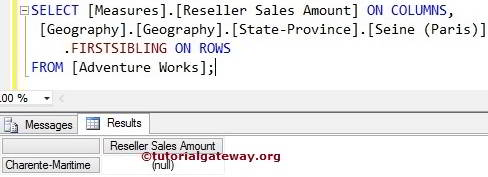# MDX FirstChild Function

The MDX FirstChild function will return the First Child member belongs to the specified member. For example, If you know a single customer name and if you want to find the Sales of a first customer, then you can use this MDX FirstChild function.

## MDX FirstChild Function Syntax

The basic syntax of the MDX FirstChild in Multidimensional Expression is as shown below:

`Member_Expression.FIRSTCHILD`

Member_Expression: Any Multidimensional Expression that returns valid Member.

For this FirstChild function in MDX query, We are going to use the below show data. The following screenshot shows the Countries inside the Geography

The following screenshot shows the [State – Provinces] inside the France Country

## MDX FirstChild Function Example

In this example, we are going to find the First Children present in the France Country. It means the first state present in the France country.

TIP: We can use MDX LastChild Function to find the Last Child Member.

```SELECT
[Measures].[Reseller Sales Amount] ON COLUMNS,
[Geography].[Geography].[Country].[France].FIRSTCHILD ON ROWS

In the above MDX Query, We used [Reseller Sales amount] on the columns

`[Measures].[Reseller Sales Amount] ON COLUMNS`

The below line of code will write the first child member of France from all the State-Provinces present in the France country.

`[Geography].[Geography].[Country].[France].FIRSTCHILD`

## MDX FirstChild Function Example 2

As we all know, Paris is one of the states in France, and we intend to find the first state present in France then, we can use this FirstChild function. In this example, we are going to find the First Children present in the State Province list and calculate the Reseller Sales Amount of that.

```SELECT
[Measures].[Reseller Sales Amount] ON COLUMNS,
[Geography].[Geography].[State-Province].[Seine (Paris)].PARENT.FIRSTCHILD ON ROWS

In the above MDX Query, We used [Reseller Sales amount] on the columns

`[Measures].[Reseller Sales Amount] ON COLUMNS`

From Below line of code,

`[Geography].[Geography].[State-Province].[Seine (Paris)].PARENT.FIRSTCHILD`

MDX will first implement the Parent function to find the parent member of a [Seine (Paris)], which is France. Please refer MDX Parent Function for further understanding

Next, It will implement FirstChild function to find the first child member of the France Country, Which is Charente-Maritime. For Charente-Maritime State, there are no sales at all. So, it is displaying Null results.

## FirstChild Function Alternative

In this example, we use the MDX FirstChild function alternative to achieve the same result. Please refer to MDX FirstSibling Function to understand the FirstSibling function.

```SELECT
[Measures].[Reseller Sales Amount] ON COLUMNS,
[Geography].[Geography].[State-Province].[Seine (Paris)].FIRSTSIBLING ON ROWS